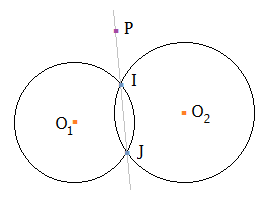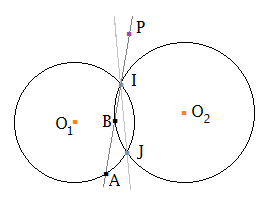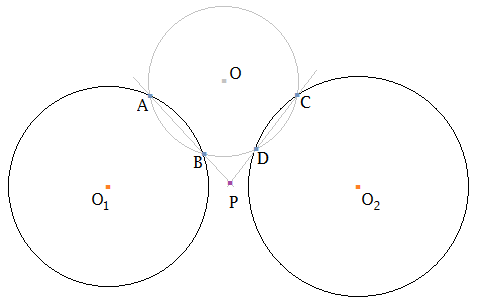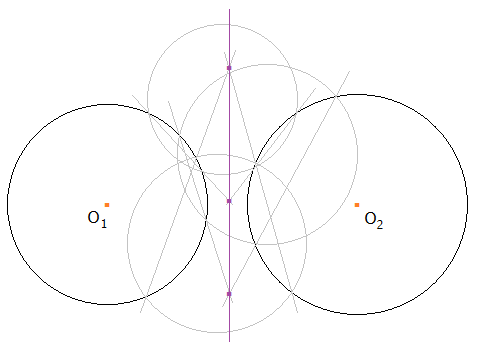## Pages

### Radical axis and radical center

In previous post, we have learned about the concept of power of a point with respect to a circle. The power of a point $P$ with respect to a circle centered at $O$ and of radius $r$ is defined by the following formula $${\cal P}(P, (O)) = \vec{PU} \times \vec{PV} = PO^2 - r^2 = (P_x - O_x)^2 + (P_y - O_y)^2 - r^2,$$ here, $U$ and $V$ are two intersection points of the circle $(O)$ with an arbitrary line passing though $P$.Power of a point: ${\cal P}(P, (O)) = \vec{PU} \times \vec{PV} = PO^2 - r^2 = (P_x - O_x)^2 + (P_y - O_y)^2 - r^2$.

The value of the power of a point gives us information about relative position of the point with respect to the circle. If the power of the point $P$ is a positive number then $P$ is outside the circle, if it is a negative number then $P$ is inside the circle, and if it is equal to zero then $P$ is on the circle.

Today, we will look at application of the power of a point concept. We will use two main tools: radical axis and radical center. Radical axis is often used to prove that a certain number of points lie on the same straight line, and radical center is used to prove a certain number of lines meet at a common point.

Suppose we have two circles $(O_1, r_1)$ and $(O_2, r_2)$ intersecting at two points $I$ and $J$ as in the following figure. If $P$ is a point on the line $IJ$ then clearly $P$ has equal power with respect to these two circles $${\cal P}(P, (O_1)) = \vec{PI} \times \vec{PJ} = {\cal P}(P, (O_2)).$$Upon more careful observation though, we see that if the point $P$ does not lie on the line $IJ$ then the powers of the point $P$ with respect to these two circles will not be the same. For instance, in the following figure, we can see that $${\cal P}(P, (O_1)) = \vec{PI} \times \vec{PA} \neq \vec{PI} \times \vec{PB} = {\cal P}(P, (O_2)).$$So we can conclude that $P$ has equal power to the two circles if and only if the point $P$ lies on the line $IJ$.

Now let us look at a different scenario. Suppose we have two circles $(O_1, r_1)$ and $(O_2, r_2)$ which do not have intersections. Is it possible to construct a point $P$ that has equal power to these two circles?

Yes, there is a simple method. Please have a ruler and a compass ready to draw with me. Let us draw a circle $(O)$ so that it cuts the circle $(O_1)$ at two points $A$, $B$, and cuts the circle $(O_2)$ at two points $C$, $D$. Let us take the intersection point $P$ of the two lines $AB$ and $CD$.As we have previously pointed out, since $P$ lies on $AB$, $P$ has equal power to $(O)$ and $(O_1)$. Since $P$ lies on $CD$, $P$ has equal power to $(O)$ and $(O_2)$. Therefore, $P$ has equal power to the two circles $(O_1)$ and $(O_2)$!

Let us now draw a lot of circles, with different positions of $(O)$, can you observe any pattern in the intersection points $P$? Can you see that $P$ is moving around on a line?We have reason now to suspect that the locus of all the points that have equal power to two circles is a straight line. This line is called the radical axis of the two circles.

Radical axis

Let us now state the theorem on radical axis. There are many ways to prove this theorem. We will show two proofs. One proof is using vector and the other makes use of coordinate system.

Theorem on radical axis. Given two circles $(O_1, r_1)$ and $(O_2, r_2)$ with two distinct centers ($O_1 \neq O_2$). Then the locus of all the point $P$ such that $P$ has equal power to the two circles is a straight line. This line is called the radical axis of the two circles $(O_1)$ and $(O_2)$. The radical axis is perpendicular to the line $O_1 O_2$ connecting the two centers.

Using the power of a point formula $${\cal P}(P, (O_1)) = PO_1^2 - r_1^2,$$ $${\cal P}(P, (O_2)) = PO_2^2 - r_2^2,$$ we can see that $${\cal P}(P, (O_1)) = {\cal P}(P, (O_2))$$ is equivalent to $$PO_2^2 - PO_1^2 = r_2^2 - r_1^2 = c.$$

A proof using vector. $$c = PO_2^2 - PO_1^2 = (\vec{PO_2} + \vec{PO_1})(\vec{PO_2} - \vec{PO_1}) = 2 \vec{PM} ~\vec{O_1 O_2},$$ here, $M$ is the midpoint of $O_1 O_2$.Draw the perpendicular line $PH$ to $O_1 O_2$, we have $$c = PO_2^2 - PO_1^2 = 2 \vec{PM} ~\vec{O_1 O_2} = 2 (\vec{PH} + \vec{HM}) \vec{O_1 O_2} = 2 \vec{HM} ~\vec{O_1 O_2}.$$
This implies that the point $H$ on $O_1 O_2$ is determined uniquely by the formula $$\overline{HM} = \frac{c}{2 \overline{O_1 O_2}}.$$
Therefore, the locus of the point $P$ is the line passing through $H$ and perpendicular to $O_1 O_2$, and the theorem is proved.

A proof using coordinate system. $$c = PO_2^2 - PO_1^2 = [(P_x - O_{2x})^2 + (P_y - O_{2y})^2] - [(P_x - O_{1x})^2 + (P_y - O_{1y})^2]$$ $$= 2(O_{1x} - O_{2x}) P_x + 2(O_{1y} - O_{2y}) P_y + (O_{2x}^2 + O_{2y}^2 - O_{1x}^2 - O_{1y}^2).$$

This means that the locus of the point $P$ is the straight line determined by the following equation $$(O_{1x} - O_{2x}) x + (O_{1y} - O_{2y}) y + \frac{1}{2}(O_{2x}^2 + O_{2y}^2 - O_{1x}^2 - O_{1y}^2 - c) = 0.$$
The gradient of this line shows that it is perpendicular to $O_1 O_2$. Thus, the theorem is proved.

We have shown two proofs of the radical axis theorem. So now we know that the locus of all points that have equal power to two circles is a straight line and this line is called the radical axis of the two circles. A radical axis is perpendicular to the line connecting the centers of the two circles. In a special case, if the two circles intersect at two points then the radical axis is the line connecting these two intersection points.

Using radical axis we can prove that certain points lie on the same straight line. The strategy is to show that each of these points has equal power to two certain circles and then conclude that they all lie on the radical axis of the two circles.

Radical center

Let us now move on to three circles. Suppose that we have three circles $(O_1)$, $(O_2)$ and $(O_3)$, and we want to find a point $P$ such that it has equal power to the three circles $${\cal P}(P, (O_1)) = {\cal P}(P, (O_2)) = {\cal P}(P, (O_3)).$$Now, if we want $P$ to have equal power to $(O_1)$ and $(O_2)$ then $P$ must be on the radical axis of the two circles $(O_1)$ and $(O_2)$. Similarly, we can see that $P$ must be on the radical axis of the two circles $(O_2)$ and $(O_3)$, and also on the radical axis of the two circles $(O_3)$ and $(O_1)$. Thus, the three radical axes concurrent at the point $P$. This point is called the radical center of the three circles $(O_1)$, $(O_2)$ and $(O_3)$.

From the radical axis theorem, we know that the radical axis is perpendicular to the line connecting the two centers of the circles. So if the centers $O_1$, $O_2$, $O_3$ are collinear then all three radical axes are perpendicular to the line $O_1 O_2 O_3$. In this case, the three radical axes are parallel.Let us state the theorem on radical center.
Theorem on radical center. Given three circles $(O_1)$, $(O_2)$ and $(O_3)$ whose centers are three distinct points.
• If the three centers $O_1$, $O_2$, $O_3$ lie on a straight line then the radical axes of the three pairs of circles $\{(O_1), (O_2)\}$, $\{(O_2), (O_3)\}$ and $\{(O_3), (O_1)\}$ are parallel to each other;
• If the three points $O_1$, $O_2$, $O_3$ are not collinear then the radical axes of the three pairs of circles $\{(O_1), (O_2)\}$, $\{(O_2), (O_3)\}$ and $\{(O_3), (O_1)\}$ meet at a single point and this point is called the radical center. The radical center has equal power to the three circles.

Application

Radical axis is a useful tool to prove the collinearity of points. To prove that a certain number of points lie on a straight line, we may try to show that each of these points has equal power to two circles and then draw the conclusion that they all lie on the radical axis of the circles.

Similarly, we can use radical center to prove the concurrency of lines. The strategy is to show that each of these lines is the radical axis of a pair of circles and then conclude that they meet at the radical center.

Let us look at some exercises.

Problem 1. Given a triangle $ABC$. Construct outside of the triangle $ABC$ three isosceles triangles $BA'C$, $CB'A$ and $AC'B$ whose bases are $BC$, $CA$ and $AB$, respectively. Prove that the lines passing through $A$, $B$, $C$, respectively, and perpendicular to $B'C'$, $C'A'$, $A'B'$ are concurrent.

Solution.Using $A'$ as the center, draw a circle passing the two points $B$ and $C$. Using $B'$ as the center, draw a circle passing the two points $C$ and $A$. And using $C'$ as the center, draw a circle passing the two points $A$ and $B$.The line passing through $A$ perpendicular to $B'C'$ clearly is the radical axis of the two circles $(B')$ and $(C')$. Similarly, the line passing through $B$ perpendicular to $C'A'$ is the radical axis of the two circles $(C')$ and $(A')$, and the line passing through $C$ perpendicular to $A'B'$ is the radical axis of the two circles $(A')$ and $(B')$. Therefore, the three lines must concurrent at the radical center of the three circles $(A')$, $(B')$, $(C')$, and the problem is proved.

Problem 2 (IMO 1995). Let $A$, $B$, $C$, $D$ be four distinct points on a line, in that order. The circles with diameters $AC$ and $BD$ intersect at $X$ and $Y$. The line $XY$ meets $BC$ at $Z$. Let $P$ be a point on the line $XY$ other than $Z$. The line $CP$ intersects the circle with diameter $AC$ at $C$ and $M$, and the line $BP$ intersects the circle with diameter $BD$ at $B$ and $N$. Prove that the lines $AM$, $DN$, $XY$ are concurrent.

Solution.To prove that $AM$, $DN$, $XY$ are concurrent, we take $T$ as the intersection point of $AM$ and $XY$ and then show that the point $T$ is on the line $DN$.

Since $T$ is on $XY$, $T$ has equal power to the two circles with diameters $BD$ and $AC$.
Since $T$ is on $AM$, $T$ has equal power to the two circles with diameters $AC$ and $AP$.
Since $T$ is on $PZ$, $T$ has equal power to the two circles with diameters $AP$ and $DP$.

It follows that $T$ has equal power to the two circles with diameters $BD$ and $DP$. The line $DN$ is the radical axis of these two circles, therefore, $T$ is on $DN$. Thus, the problem is proved.

Today we have learned about the power of a point with respect to a circle and the concept of radical axis and radical center of circles. Let us stop here for now. In the next post we will use this tool to prove Pascal's theorem for the circle case. Hope to see you there.

Homework.

1. Use Pythagorean Theorem to find the locus of all the points $P$ such that $$PO_2^2 - PO_1^2 = c.$$ (Hint: Draw the line $PH$ perpendicular to $O_1 O_2$ and then use Pythagorean Theorem.)

2. Suppose that $A_1 A_2$, $B_1 B_2$, $C_1 C_2$, $D_1 D_2$ are tangent to the two circles $(O_1)$ and $(O_2)$ as in the following figure. Prove that the midpoints $A$, $B$, $C$, $D$ of $A_1 A_2$, $B_1 B_2$, $C_1 C_2$, $D_1 D_2$ lie on a straight line.3. Given a square $ABCD$, using its four vertices as center, draw four circles of equal radius. Take a point $P$ lying outside of these circles. Through $P$ draw two lines such that the first line intersects with the circle $(A)$ at two points $A_1$, $A_2$, and intersects with the circle $(C)$ at two points $C_1$, $C_2$; the second line intersects with the circle $(B)$ at two points $B_1$, $B_2$, and intersects with the circle $(D)$ at two points $D_1$, $D_2$. Prove that $$PA_1 \times PA_2 + PC_1 \times PC_2 = PB_1 \times PB_2 + PD_1 \times PD_2.$$4. (IMO 2000). Suppose $AB$ is tangent to the circles $CAMN$ and $NMBD$, $M$ lies between $C$ and $D$ on the line $CD$, and $CD$ is parallel to $AB$. The chords $NA$ and $CM$ meet at $P$; the chords $NB$ and $MD$ meet at $Q$. The rays $CA$ and $DB$ meet at $E$. Prove that $PE = QE$.Hint:
• Prove that $NM$ intersects $AB$ at the midpoint of $AB$
• Prove that $M$ is the midpoint of $PQ$
• Prove that $AB$ is a midline of the triangle $ECD$
• Prove that $EM$ is perpendicular to $CD$
• Prove that $EPQ$ is an isosceles triangle